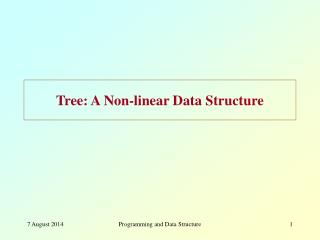DownloadDownload PresentationTree: A Non-linear Data Structure

# Tree: A Non-linear Data Structure

Télécharger la présentation## Tree: A Non-linear Data Structure

- - - - - - - - - - - - - - - - - - - - - - - - - - - E N D - - - - - - - - - - - - - - - - - - - - - - - - - - -
##### Presentation Transcript

1. Tree: A Non-linear Data Structure Programming and Data Structure

2. Illustration 50 Level 1 Level 2 30 75 Level 3 12 45 53 80 20 35 48 78 85 Level 4 Programming and Data Structure

3. Binary Tree • A new data structure • Binary means two. • Definition • A binary tree is either empty, or it consists of a node called the root, together with two binary trees called the left subtree and the right subtree. Root Left subtree Right subtree Programming and Data Structure

4. Examples of Binary Trees Programming and Data Structure

5. Not Binary Trees Programming and Data Structure

6. How to Implement a Binary Tree? • Two pointers in every node (left and right). struct nd { int element; struct nd *lptr; struct nd *rptr; }; typedef nd node; node *root; /* Will point to the root of the tree */ Programming and Data Structure

7. An Example a • Create the tree a = (node *) malloc (sizeof (node)); b = (node *) malloc (sizeof (node)); c = (node *) malloc (sizeof (node)); d = (node *) malloc (sizeof (node)); a->element = 10; a->lptr = b; a->rptr = c; b->element = 5; b->lptr = NULL; b->rptr = NULL; c->element = 20; c->lptr = d; c->rptr = NULL; d->element = 15; d->lptr = NULL; d->rptr = NULL; root = a; 10 b c 5 20 15 d Programming and Data Structure

8. Traversal of Binary Trees • In many applications, it is required to move through all the nodes of a binary tree, visiting each node in turn. • For n nodes, there exists n! different orders. • Three traversal orders are most common: • Inorder traversal • Preorder traversal • Postorder traversal Programming and Data Structure

9. Inorder Traversal • Recursively, perform the following three steps: • Visit the left subtree. • Visit the root. • Visit the right subtree. LEFT-ROOT-RIGHT Programming and Data Structure

10. Example:: inorder traversal 10 20 30 10 20 30 40 25 50 60 20 10 30 40 20 25 10 50 30 60 Programming and Data Structure

11. a b c d e f g h i j k . d g b . a h e j i k c f Programming and Data Structure

12. Preorder Traversal • Recursively, perform the following three steps: • Visit the root. • Visit the left subtree. • Visit the right subtree. ROOT-LEFT-RIGHT Programming and Data Structure

13. 10 20 30 40 25 50 60 Example:: preorder traversal 10 20 30 10 20 30 10 20 40 25 30 50 60 Programming and Data Structure

14. a b c d e f g h i j k a b d . g . c e h i j k f Programming and Data Structure

15. Postorder Traversal • Recursively, perform the following three steps: • Visit the left subtree. • Visit the right subtree. • Visit the root. LEFT-RIGHT-ROOT Programming and Data Structure

16. Example:: postorder traversal 10 20 30 10 20 30 40 25 50 60 20 30 10 40 25 20 50 60 30 10 Programming and Data Structure

17. a b c d e f g h i j k . g d . b h j k i e f c a Programming and Data Structure

18. Implementations void inorder (node *root) { if (root != NULL) { inorder (root->left); printf (“%d “, root->element); inorder (root->right); } } void preorder (node *root) { if (root != NULL) { printf (“%d “, root->element); inorder (root->left); inorder (root->right); } } void postorder (node *root) { if (root != NULL) { inorder (root->left); inorder (root->right); printf (“%d “, root->element); } } Programming and Data Structure

19. Graph :: another important data structure • Definition • A graph G={V,E} consists of a set of vertices V, and a set of edges E which connect pairs of vertices. • If the edges are undirected, G is called an undirected graph. • If at least one edge in G is directed, it is called a directed graph. Programming and Data Structure

20. How to represent a graph in a program? • Many ways • Adjacency matrix • Incidence matrix, etc. Programming and Data Structure

21. Adjacency Matrix e1 e5 1 2 6 e2 e4 e6 e7 e3 e8 3 4 5 . 1 2 3 4 5 6 1 0 1 1 0 0 0 2 1 0 0 1 1 1 3 1 0 0 1 0 0 4 0 1 1 0 1 0 5 0 1 0 1 0 1 6 0 1 0 0 1 0 Programming and Data Structure

22. Incidence Matrix e1 e5 1 2 6 e2 e4 e6 e7 e3 e8 3 4 5 . e1 e2 e3 e4 e5 e6 e7 e8 1 1 1 0 0 0 0 0 0 2 1 0 0 1 1 1 0 0 3 0 1 1 0 0 0 0 0 4 0 0 1 1 0 0 0 1 5 0 0 0 0 0 1 1 1 6 0 0 0 0 1 0 1 0 Programming and Data Structure